• MATLAB多元线性回归: 基于MATLAB多元非线性回归模型.pdf 多元线性回归建模以及MATLAB和SPSS求解.pdf MATLAB语言在多元线性回归中的应用.pdf
• matlab多元回归工具箱 Excel数据分析工具进行多元回归分析.docmatlab多元回归工具箱 Excel数据分析工具进行多元回归分析导读：就爱阅读网友为您分享以下“Excel数据分析工具进行多元回归分析”资讯，希望对您有所...

matlab多元回归工具箱 Excel数据分析工具进行多元回归分析.doc

matlab多元回归工具箱 Excel数据分析工具进行多元回归分析

导读：就爱阅读网友为您分享以下“Excel数据分析工具进行多元回归分析”资讯，希望对您有所帮助，感谢您对92的支持!

使用Excel 数据分析工具进行多元回归分析

使用Excel 数据分析工具进行多元回归分析与简单的回归估算分析方法基本相同。但是由于有些电脑在安装办公软件时并未加载数据分析工具，所以从加载开始说起(以Excel2010版为例，其余版本都可以在相应界面找到)。

点击“文件”，如下图：

在弹出的菜单中选择“选项”，如下图所示：

在弹出的“选项”菜单中选择“加载项”，在“加载项”多行文本框中使用滚动条找到并选中“分析工具库”，然后点击最下方的“转到”，如下图所示：

在弹出的“加载宏”菜单中选择“分析工具库”，然后点击 “确定”，如下图所示：

加载完毕，在“数据”工具栏中就出现“数据分析”工具库，如下图所示：

给出原始数据，自变量的值在A2：I21单元格区间中，因变量的值在J2：J21中，如下图所示：

假设回归估算表达式为：

试使用Excel 数据分析工具库中的回归分析工具对其回归系数进行估算并进行回归分析： 点击“数据”工具栏中中的“数据分析”工具库，如下图所示：

在弹出的“数据分析”-“分析工具”多行文本框中选择“回归”，然后点击 “确定”，如下图所示：

弹出“回归”对话框并作如下图的选择：

上述选择的具体方法是：

在“Y 值输入区域”，点击右侧折叠按钮，选取函数Y 数据所在单元格区域J2：J21，选完后再单击折叠按钮返回；这过程也可以直接在“Y 值输入区域”文本框中输入J2：J21；

在“X 值输入区域”，点击右侧折叠按钮，选取自变量数据所在单元格区域A2：I21，选完后再单击折叠按钮返回；这过程也可以直接在“X 值输入区域”文本框中输入A2：I21； 置信度可选默认的95%。

在“输出区域”如选“新工作表”，就将统计分析结果输出到在新表内。为了比较对照，我选本表内的空白区域，左上角起始单元格为K10. 点击确定后，输出结果如下：

第一张表是“回归统计表”(K12：L17):

其中：

Multiple R ：(复相关系数R )R 2的平方根，又称相关系数，用来衡量自变量x 与y 之间的相关程度的大小。本例R=0.9134表明它们之间的关系为高度正相关。(Multiple ：复合、多种) R Square：复测定系数，上述复相关系数R 的平方。用来说明自变量解释因变量y 变差的程度，以测定因变量y 的拟合效果。此案例中的复测定系数为0.8343，表明用用自变量可解释因变量变差的83.43%

标准误差：用来衡量拟合程度的大小，也用于计算与回归相关的其它统计量，此值越小，说明拟合程度越好

观察值：用于估计回归方程的数据的观察值个数。

第二张表是“方差分析表”：主要作用是通过F 检验来判定回归模型的回归效果。

该案例中的Significance F(F 显著性统计量)的P 值为0.00636，小于显著性水平0.05，所以说该回归方程回归效果显著，方程中至少有一个回归系数显著不为0. (Significance ：显著) 第三张表是“回归参数表”：

K26：K35为常数项和b1～b9的排序默认标示。

L26：L35为常数项和b1～b9的值，据此可得出估算的回归方程为：

该表中重要的是O 列, 该列的O26：O35中的 P-value为回归系数t 统计量的P 值。

值得注意的是：其中b1、b7的t 统计量的P 值为0.0156和0.0175，远小于显著性水平0.05，因此该两项的自变量与y 相关。而其他各项的t 统计量的P 值远大于b1、b7的t 统计量的P 值，但如此大的P 值说明这些项的自变量与因变量不存在相关性，因此这些项的回归系数不显著。

回归分析是一种应用很广的数量分析方法，用于分析事物间的统计关系，侧重数量关系变化。回归分析在数据分析中占有比较重要的位置。

一元线性回归模型：指只有一个解释变量的线性回归模型，用来揭示被解释变量与另一个解释变量的线性关系。

多元线性回归模型：指含有多个揭示变量的线性回归模型，用来揭示被解释变量与多个解释变量的线性关系。

此篇文章主要讲述多元线性回归分析。

方法/步骤

线性回归分析的内容比较多，比如回归方程的拟合优度检验、回归方程的显著性检验、回归系数的显著性检验、残差分析、变量的筛选问题、变量的多重共线性问题。

操作见图。回归分析通常需要

展开全文• 主要用于数学建模（Matlab）的学习，下载下来换成你的数据就可以用了。
• 《第11讲_matlab多元回归分析》由会员分享，可在线阅读，更多相关《第11讲_matlab多元回归分析(53页珍藏版)》请在人人文库网上搜索。1、,数学建模与数学实验,回归分析,.,实验目的,实验内容,1回归分析的基本理论.,3...

《第11讲_matlab多元回归分析》由会员分享，可在线阅读，更多相关《第11讲_matlab多元回归分析(53页珍藏版)》请在人人文库网上搜索。

1、,数学建模与数学实验,回归分析,.,实验目的,实验内容,1回归分析的基本理论.,3实验作业.,2用数学软件求解回归分析问题.,.,一元线性回归,多元线性回归,回归分析,数学模型及定义,*模型参数估计,*检验、预测与控制,可线性化的一元非线 性回归(曲线回归),数学模型及定义,*模型参数估计,逐步回归分析,* 多元线性回归中的 检验与预测,.,一、数学模型,例1 测16名成年女子的身高与腿长所得数据如下：,以身高x为横坐标，以腿长y为纵坐标将这些数据点(xi，yi)在平面直角坐标系上标出.,散点图,解答,.,一元线性回归分析的主要任务是：,返回,.,二、模型参数估计,1回归系数的最小二乘估计,.。

2、,.,返回,.,三、检验、预测与控制,1回归方程的显著性检验,.,()F检验法,()t 检验法,.,()r 检验法,.,2回归系数的置信区间,.,3预测与控制,(1)预测,.,(2)控制,返回,.,四、可线性化的一元非线性回归 (曲线回归),例2 出钢时所用的盛钢水的钢包，由于钢水对耐火材料的侵蚀， 容积不断增大.我们希望知道使用次数与增大的容积之间的关 系.对一钢包作试验，测得的数据列于下表：,解答,.,散 点 图,此即非线性回归或曲线回归,问题(需要配曲线),配曲线的一般方法是：,.,通常选择的六类曲线如下：,返回,.,一、数学模型及定义,返回,.,二、模型参数估计,.,返回,.,三、多元。

3、线性回归中的检验与预测,()F 检验法,()r 检验法,(残差平方和),.,2预测,(1)点预测,(2)区间预测,返回,.,四、逐步回归分析,(4)“有进有出”的逐步回归分析.,(1)从所有可能的因子(变量)组合的回归方程中选择最优者；,(2)从包含全部变量的回归方程中逐次剔除不显著因子；,(3)从一个变量开始，把变量逐个引入方程；,选择“最优”的回归方程有以下几种方法：,“最优”的回归方程就是包含所有对Y有影响的变量, 而不包含对Y影响不显著的变量回归方程.,以第四种方法，即逐步回归分析法在筛选变量方面较为理想.,.,这个过程反复进行，直至既无不显著的变量从回归方程中剔除，又无显著变量可引入。

4、回归方程时为止.,逐步回归分析法的思想：,从一个自变量开始，视自变量Y对作用的显著程度，从大到小地依次逐个引入回归方程.,当引入的自变量由于后面变量的引入而变得不显著时，要将其剔除掉.,引入一个自变量或从回归方程中剔除一个自变量，为逐步回归的一步.,对于每一步都要进行Y值检验，以确保每次引入新的显著性变量前回归方程中只包含对Y作用显著的变量.,返回,.,统计工具箱中的回归分析命令,1多元线性回归,2多项式回归,3非线性回归,4逐步回归,返回,.,多元线性回归,b=regress( Y, X ),1确定回归系数的点估计值：,.,3画出残差及其置信区间： rcoplot(r，rint),2求回归系。

5、数的点估计和区间估计、并检验回归模型： b, bint,r,rint,stats=regress(Y,X,alpha),.,例1,解：,1输入数据： x=143 145 146 147 149 150 153 154 155 156 157 158 159 160 162 164; X=ones(16,1) x; Y=88 85 88 91 92 93 93 95 96 98 97 96 98 99 100 102;,2回归分析及检验： b,bint,r,rint,stats=regress(Y,X) b,bint,stats,To MATLAB(liti11),题目,.,3残差分析，作残差图。

6、： rcoplot(r,rint),从残差图可以看出，除第二个数据外，其余数据的残差离零点均较近，且残差的置信区间均包含零点，这说明回归模型 y=-16.073+0.7194x能较好的符合原始数据，而第二个数据可视为异常点.,4预测及作图： z=b(1)+b(2)* plot(x,Y,k+,x,z,r),返回,To MATLAB(liti12),.,多 项 式 回 归,(一)一元多项式回归,y=a1xm+a2xm-1+amx+am+1,.,法一,直接作二次多项式回归： t=1/30:1/30:14/30; s=11.86 15.67 20.60 26.69 33.71 41.93 51.13 。

7、61.49 72.90 85.44 99.08 113.77 129.54 146.48; p,S=polyfit(t,s,2),To MATLAB(liti21),得回归模型为 ：,.,法二,化为多元线性回归： t=1/30:1/30:14/30; s=11.86 15.67 20.60 26.69 33.71 41.93 51.13 61.49 72.90 85.44 99.08 113.77 129.54 146.48; T=ones(14,1) t (t.2); b,bint,r,rint,stats=regress(s,T); b,stats,To MATLAB(liti22),得回。

8、归模型为 ：,Y=polyconf(p,t,S) plot(t,s,k+,t,Y,r),预测及作图,To MATLAB(liti23),.,(二)多元二项式回归,命令：rstool(x，y，model, alpha),.,例3 设某商品的需求量与消费者的平均收入、商品价格的统计数 据如下，建立回归模型，预测平均收入为1000、价格为6时 的商品需求量.,法一,直接用多元二项式回归： x1=1000 600 1200 500 300 400 1300 1100 1300 300; x2=5 7 6 6 8 7 5 4 3 9; y=100 75 80 70 50 65 90 100 110 60。

9、; x=x1 x2; rstool(x,y,purequadratic),.,在画面左下方的下拉式菜单中选”all”, 则betarmse和residuals都传送到MATLAB工作区中.,将左边图形下方方框中的“800”改成1000，右边图形下方的方框中仍输入6.则画面左边的“Predicted Y”下方的数据由原来的“86.3791”变为88.4791，即预测出平均收入为1000价格为6时的商品需求量为88.4791.,.,在MATLAB工作区中输入命令： beta, rmse,To MATLAB(liti31),.,结果为: b = 110.5313 0.1464 -26.5709 -0。

10、.0001 1.8475 stats = 0.9702 40.6656 0.0005,法二,To MATLAB(liti32),返回,.,非线性回 归,(1)确定回归系数的命令： beta，r，J=nlinfit(x,y,model,beta0),(2)非线性回归命令：nlintool(x，y，model, beta0，alpha),1回归：,.,例 4 对第一节例2，求解如下：,2输入数据： x=2:16; y=6.42 8.20 9.58 9.5 9.7 10 9.93 9.99 10.49 10.59 10.60 10.80 10.60 10.90 10.76; beta0=8 2;,3。

11、求回归系数： beta,r ,J=nlinfit(x,y,volum,beta0)； beta,得结果：beta = 11.6036 -1.0641,即得回归模型为：,To MATLAB(liti41),题目,.,4预测及作图： YY,delta=nlpredci(volum,x,beta,r ,J)； plot(x,y,k+,x,YY,r),To MATLAB(liti42),.,例5 财政收入预测问题：财政收入与国民收入、工业总产值、农业总产值、总人口、就业人口、固定资产投资等因素有关.表中列出了19521981年的原始数据，试构造预测模型.,解 设国民收入、工业总产值、农业总产值、总人口。

12、、就业人口、固定资产投资分别为x1、x2、x3、x4、x5、x6，财政收入为y，设变量之间的关系为： y= ax1+bx2+cx3+dx4+ex5+fx6 使用非线性回归方法求解.,.,1 对回归模型建立M文件model.m如下: function yy=model(beta0,X) a=beta0(1); b=beta0(2); c=beta0(3); d=beta0(4); e=beta0(5); f=beta0(6); x1=X(:,1); x2=X(:,2); x3=X(:,3); x4=X(:,4); x5=X(:,5); x6=X(:,6); yy=a*x1+b*x2+c*x3+d。

13、*x4+e*x5+f*x6;,.,2. 主程序liti6.m如下:,X=598.00 349.00 461.00 57482.00 20729.00 44.00 . 2927.00 6862.00 1273.00 100072.0 43280.00 496.00; y=184.00 216.00 248.00 254.00 268.00 286.00 357.00 444.00 506.00 . 271.00 230.00 266.00 323.00 393.00 466.00 352.00 303.00 447.00 . 564.00 638.00 658.00 691.00 655.00 。

14、692.00 657.00 723.00 922.00 . 890.00 826.00 810.0; beta0=0.50 -0.03 -0.60 0.01 -0.02 0.35; betafit = nlinfit(X,y,model,beta0),To MATLAB(liti6),.,betafit = 0.5243 -0.0294 -0.6304 0.0112 -0.0230 0.3658 即y= 0.5243x1-0.0294x2-0.6304x3+0.0112x4-0.0230 x5+0.3658x6,结果为:,返 回,.,逐 步 回 归,逐步回归的命令是： stepwise(x，y。

15、，inmodel，alpha),运行stepwise命令时产生三个图形窗口：Stepwise Plot，Stepwise Table，Stepwise History.,在Stepwise Plot窗口，显示出各项的回归系数及其置信区间.,Stepwise Table 窗口中列出了一个统计表，包括回归系数及其置信区间，以及模型的统计量剩余标准差(RMSE)、相关系数(R-square)、F值、与F对应的概率P.,.,例6 水泥凝固时放出的热量y与水泥中4种化学成分x1、x2、x3、 x4 有关，今测得一组数据如下，试用逐步回归法确定一个 线性模 型.,1数据输入： x1=7 1 11 11 7。

16、 11 3 1 2 21 1 11 10; x2=26 29 56 31 52 55 71 31 54 47 40 66 68; x3=6 15 8 8 6 9 17 22 18 4 23 9 8; x4=60 52 20 47 33 22 6 44 22 26 34 12 12; y=78.5 74.3 104.3 87.6 95.9 109.2 102.7 72.5 93.1 115.9 83.8 113.3 109.4; x=x1 x2 x3 x4;,.,2逐步回归： (1)先在初始模型中取全部自变量： stepwise(x,y) 得图Stepwise Plot 和表Stepwise T。

17、able,图Stepwise Plot中四条直线都是虚线，说明模型的显著性不好,从表Stepwise Table中看出变量x3和x4的显著性最差.,.,(2)在图Stepwise Plot中点击直线3和直线4，移去变量x3和x4,移去变量x3和x4后模型具有显著性.,虽然剩余标准差(RMSE)没有太大的变化，但是统计量F的 值明显增大，因此新的回归模型更好.,To MATLAB(liti51),.,(3)对变量y和x1、x2作线性回归： X=ones(13,1) x1 x2; b=regress(y,X),得结果：b = 52.5773 1.4683 0.6623 故最终模型为：y=52.5773+1.4683x1+0.6623x2,To MATLAB(liti52),返回,.,作 业,.,.,4混凝土的抗压强度随养护时间的延长而增加，现将一批混凝土作成12个试块，记录了养护日期x(日)及抗压强度y(kg/cm2)的数据：,.,谢谢大家。

展开全文• 多元线性回归:在回归分析中，如果有两个或两个以上的自变量，就称为多元回归。事实上，一种现象常常是与多个因素相联系的，由多个自变量的最优组合共同来预测或估计因变量，比只用一个自变量进行预测或估计更有效，...测绘工程
• 2 2 Matlab 实现多元回归实例 一一般多元回归 一般在生产实践和科学研究中人们得到了参数 x ?x1,xn ? 和因变量 y 的数据需要求出关系式 y ? f ? x ? 这时就可以用到回归分析的方法如果 只考虑 f 是线性函数的情形当...
• 利用matlab实现回归分析作业，可以作为数学和计算机相关专业的作业
• 本程序是主成分回归的一个详细举例，有注释，方便做主成分回归分析的人参考。
• 文章目录关于多元回归分析应用范围举例结果分析例题Matlab代码 关于多元回归分析 应用范围举例 结果分析 例题 Matlab代码 在桌面创建txt文件并输入： 1 135.0 13.70 12.68 80.32 0.16 16 102.5 17.48 ...

关于多元回归分析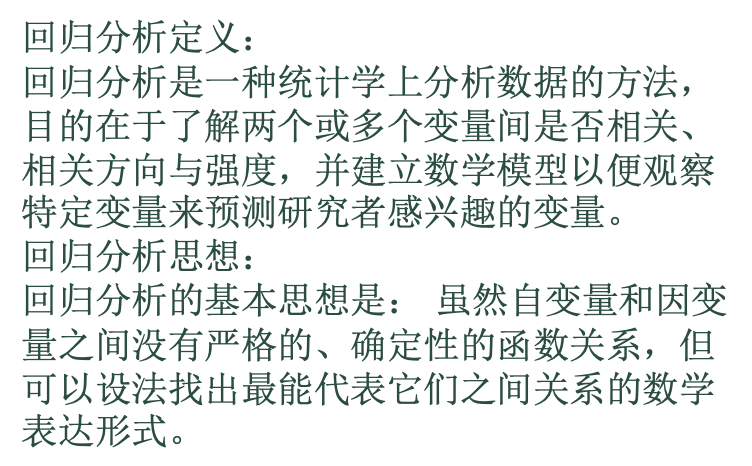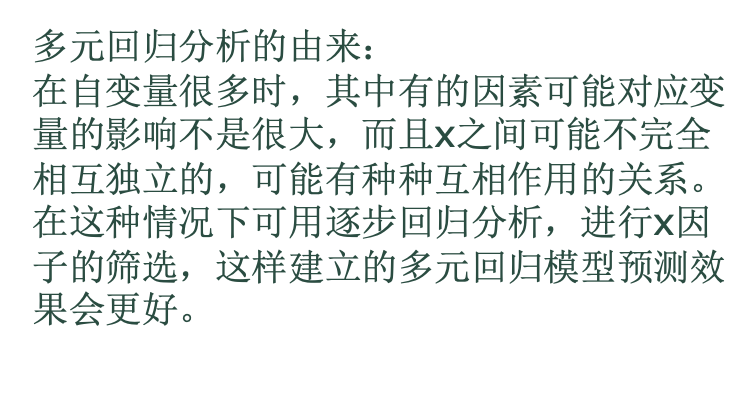应用范围举例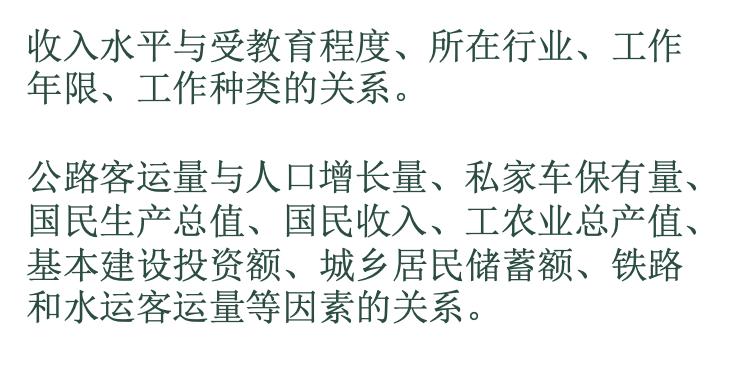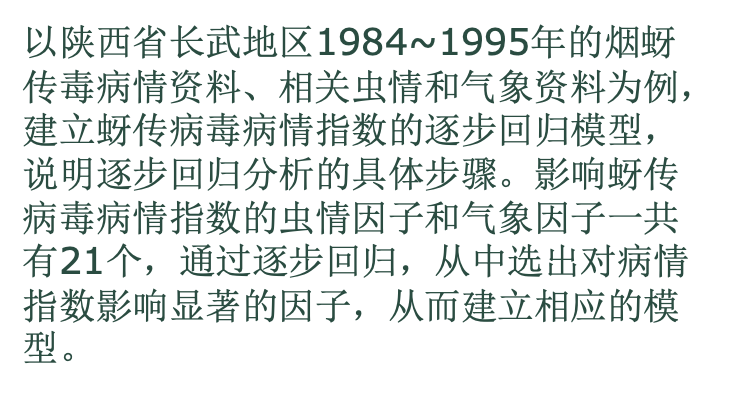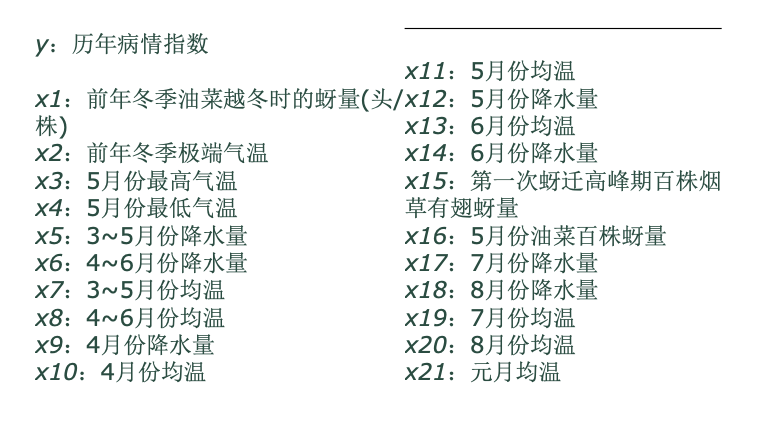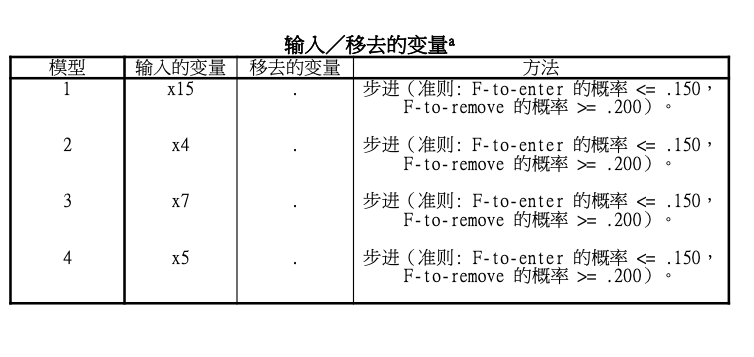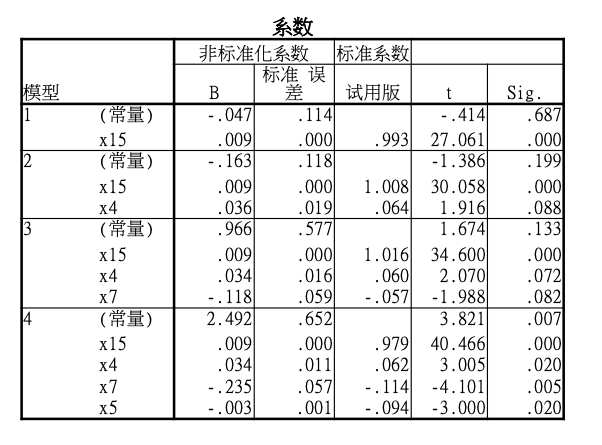结果分析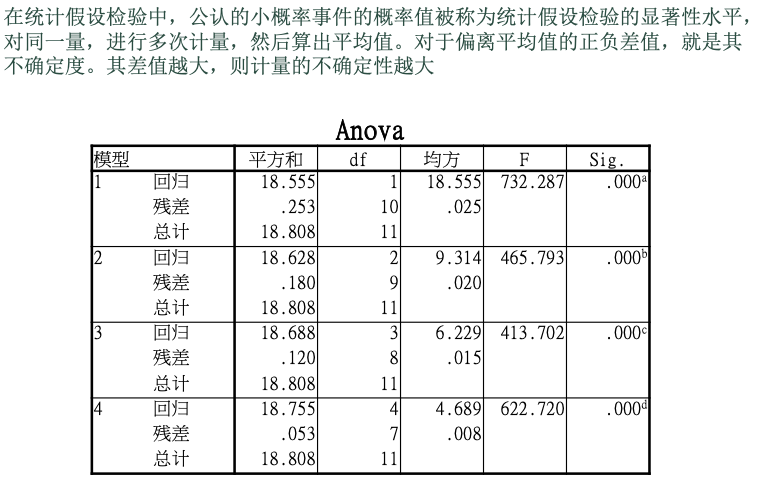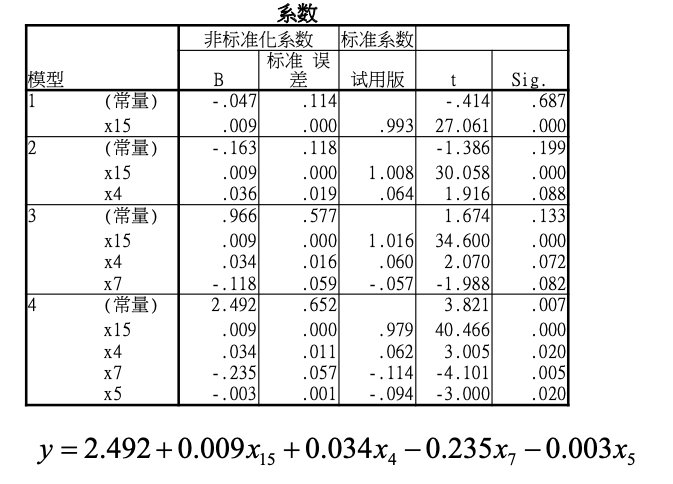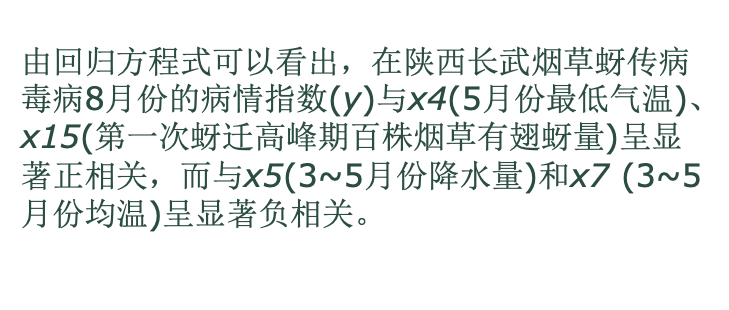例题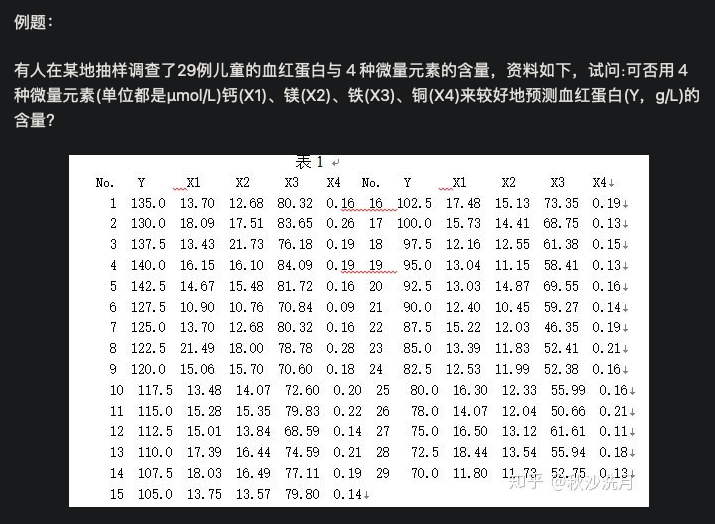Matlab代码

在桌面创建txt文件并输入：

1 135.0 13.70 12.68 80.32 0.16 16 102.5 17.48 15.13 73.35 0.19

2 130.0 18.09 17.51 83.65 0.26 17 100.0 15.73 14.41 68.75 0.13

3 137.5 13.43 21.73 76.18 0.19 18 97.5 12.16 12.55 61.38 0.15

4 140.0 16.15 16.10 84.09 0.19 19 95.0 13.04 11.15 58.41 0.13

5 142.5 14.67 15.48 81.72 0.16 20 92.5 13.03 14.87 69.55 0.16

6 127.5 10.90 10.76 70.84 0.09 21 90.0 12.40 10.45 59.27 0.14

7 125.0 13.70 12.68 80.32 0.16 22 87.5 15.22 12.03 46.35 0.19

8 122.5 21.49 18.00 78.78 0.28 23 85.0 13.39 11.83 52.41 0.21

9 120.0 15.06 15.70 70.60 0.18 24 82.5 12.53 11.99 52.38 0.16

10 117.5 13.48 14.07 72.60 0.20 25 80.0 16.30 12.33 55.99 0.16

11 115.0 15.28 15.35 79.83 0.22 26 78.0 14.07 12.04 50.66 0.21

12 112.5 15.01 13.84 68.59 0.14 27 75.0 16.50 13.12 61.61 0.11

13 110.0 17.39 16.44 74.59 0.21 28 72.5 18.44 13.54 55.94 0.18

14 107.5 18.03 16.49 77.11 0.19 29 70.0 11.80 11.73 52.75 0.13

代码：

clc ,clear

n=29;m=4;

x=[q(:,1:6);15 105.0 13.75 13.57 79.80 0.14;q(:,7:12);]

y=[x(:,2)];

x1=[x(:,3)];

x2=[x(:,4)];

x3=[x(:,5)];

x4=[x(:,6)];

X=[ones(n,1),x1,x2,x3,x4]

[b,bint,r,rint,s]=regress(y,X);

s2=sum(r.^2)/(n-m-1);

b,bint,s,s2

rcoplot(r,rint)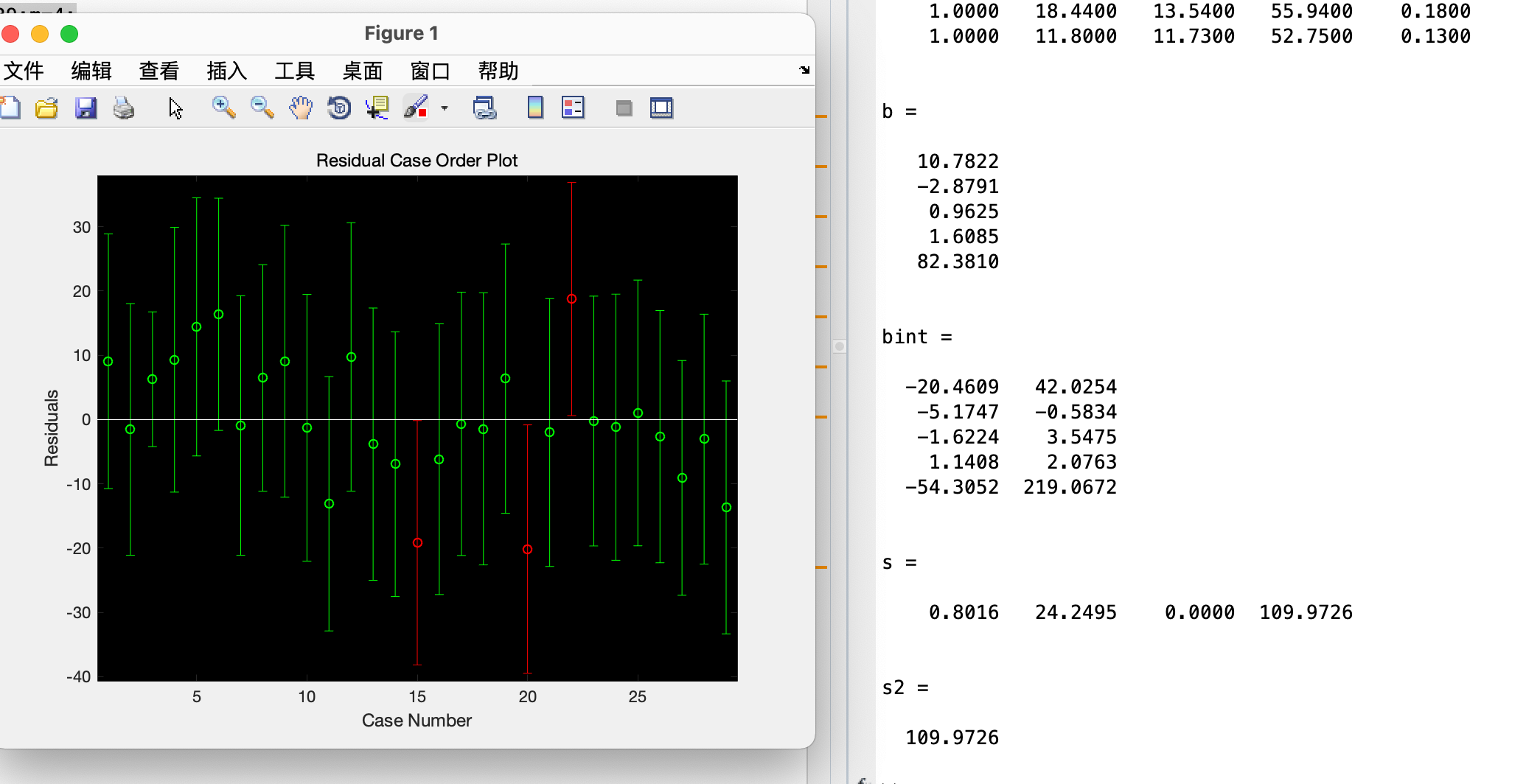得到残差图，此时可见第15与第20、22点是异常点，于是删除上述三点，对其进行优化，再次进行回归得到改进后的回归模型的系数、系数置信区间与统计量。

clc ,clear

n=26;m=4;

q(1:4,7:12)

q(6,7:12)

q(8:14,7:12)

q1=[q(1:4,7:12);q(6,7:12);q(8:14,7:12)]

x=[q(:,1:6);q1;]

y=[x(:,2)];

x1=[x(:,3)];

x2=[x(:,4)];

x3=[x(:,5)];

x4=[x(:,6)];

X=[ones(n,1),x1,x2,x3,x4]

[b,bint,r,rint,s]=regress(y,X);

s2=sum(r.^2)/(n-m-1);

b,bint,s,s2

rcoplot(r,rint)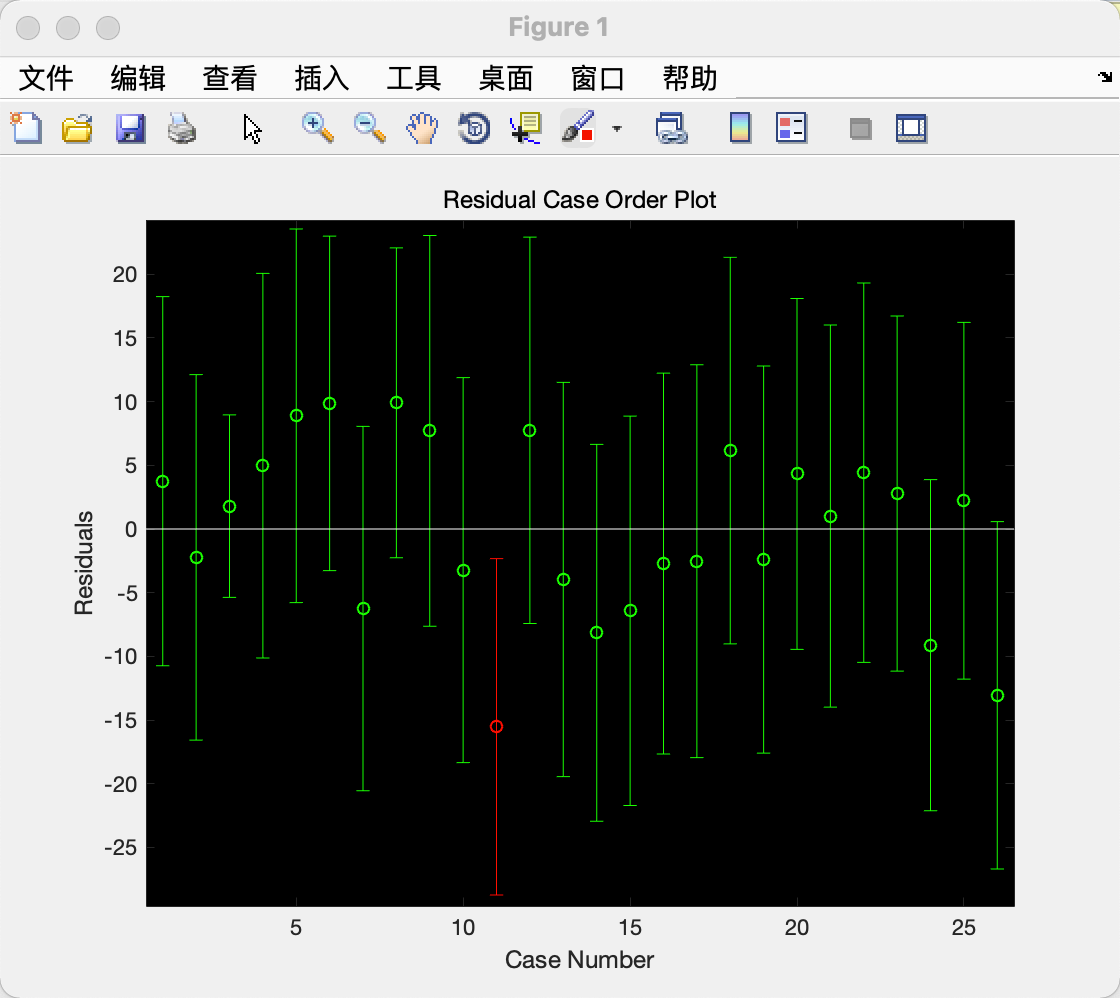展开全文• 基于matlab的多元线性回归分析

万次阅读 多人点赞 2020-04-23 14:41:29
在社会生活及生产实践中会经常遇到一种...知道了这些，我们就可以对该量变化所反映的相关问题做出分析和评价，并对其未来发展趋势进行预测和控制，这里就要用到数理统计中一个非常重要而普遍的分析方法，即回归分析法。

二、多元线性回归原理

2.1、数学模型

在社会生活及生产实践中会经常遇到一种问题，即我们非常关注一个量的变化，而这个量受到另一个或是多个因素的影响，我们想要了解这些因素是如何影响我们最为关注的这个量的以及这些因素对我们最为关注的这个量的影响权重分别有多大，知道了这些，我们就可以对该量变化所反映的相关问题做出分析和评价，并对其未来发展趋势进行预测和控制，这里就要用到数理统计中一个非常重要而普遍的分析方法，即回归分析法。

如果一个因变量y与k个自变量 x 1 , x 2 , … , x k x_{1}, x_{2}, \dots, x_{k} 存在线性相关关系，那么就可以用多元线性回归模型
y = a 0 + a 1 x 1 + a 2 x 2 + … + a k x k , 公式1 y=a_{0}+a_{1} x_{1}+a_{2} x_{2}+\ldots+a_{k} x_{k},\text{公式1}
对其进行描述，其中未知常量 a 0 , a 1 , … , a k a_{0}, a_{1}, \dots, a_{k} 称为回归模型系数，若n次抽样，第 i i 次抽样数据为 ( y i , x 1 i , x 2 i , ⋯   , x k i ) \left(y_{i}, x_{1 i}, x_{2 i}, \cdots, x_{k i}\right) 那么就有
{ y 1 = a 0 + a 1 x 11 + a 2 x 21 + … + a k x k 1 + ε 1 y 2 = a 0 + a 1 x 12 + a 2 x 22 + … + a k x k 2 + ε 2 ⋮ y n = a 0 + a 1 x 1 i + a 2 x 2 i + … + a i x k i + ε n 公式2 \left\{\begin{array}{l}y_{1}=a_{0}+a_{1} x_{11}+a_{2} x_{21}+\ldots+a_{k} x_{k1}+\varepsilon_{1} \\ y_{2}=a_{0}+a_{1} x_{12}+a_{2} x_{22}+\ldots+a_{k} x_{k2}+\varepsilon_{2} \\ \vdots \\ y_{n}=a_{0}+a_{1} x_{1 i}+a_{2} x_{2 i}+\ldots+a_{i} x_{ki}+\varepsilon_{n}\end{array}\right.\text{公式2}
其中 ε 0 , ε 1 , … , ε n \varepsilon_{0}, \varepsilon_{1}, \ldots, \varepsilon_{n} 为随机误差项，回归分析的主要任务就是以误差 ε 0 , ε 1 , … , ε n \varepsilon_{0}, \varepsilon_{1}, \ldots, \varepsilon_{n} 的平方和最小为原则，求多元回归模型的回归系数 a 0 , a 1 , … , a k a_{0}, a_{1}, \dots, a_{k}

求解这个方程是要以 S = ∑ i = 1 i = n ε i 2 = ∑ i = 1 i = n ( a 0 + a 1 x 1 i + ⋯ + a k x k i − y i ) 2 S=\sum_{i=1}^{i=n} \varepsilon_{i}^{2}=\sum_{i=1}^{i=n}\left(a_{0}+a_{1} x_{1 i}+\dots+a_{k} x_{k i}-y_{i}\right)^{2} 为最小原则，求 a 0 , a 1 , … , a k a_{0}, a_{1}, \dots, a_{k} 要使得S最小，应该满足 ∂ S ∂ a j = 0 , j = 0 , 1 , … , k \frac{\partial S}{\partial a_{j}}=0, j=0,1,\ldots,k
即 { ∑ i = 1 i = n 2 ( a 0 + a 1 x 1 i + a 2 x 2 i + ⋯ + a k x k i − y i ) = 0 ∑ i = 1 i = n 2 ( a 0 + a 1 x 1 i + a 2 x 2 i + ⋯ + a k x k i − y i ) x 1 i = 0 ⋮ ∑ i = 1 i = n 2 ( a 0 + a 1 x 1 i + a 2 x 2 i + ⋯ + a k x k i − y i ) x n i = 0 , 公式3 即\left\{\begin{array}{l}\sum_{i=1}^{i=n} 2\left(a_{0}+a_{1} x_{1 i}+a_{2} x_{2 i}+\dots+a_{k} x_{k i}-y_{i}\right)=0 \\ \sum_{i=1}^{i=n} 2\left(a_{0}+a_{1} x_{1 i}+a_{2} x_{2 i}+\dots+a_{k} x_{k i}-y_{i}\right) x_{1 i}=0 \\ \vdots \\ \sum_{i=1}^{i=n} 2\left(a_{0}+a_{1} x_{1 i}+a_{2} x_{2 i}+\dots+a_{k} x_{k i}-y_{i}\right) x_{n i}=0\end{array}\right.,\text{公式3}

有 { n a 0 + ∑ i = 1 i = n x 1 i a 1 + ⋯ + ∑ i = 1 i = n x k i a k = ∑ i = 1 i = n y i ∑ i = 1 i = n x 1 i a 0 + ∑ i = 1 i = n x 1 i 2 a 1 + ⋯ + ∑ i = 1 i = n x 11 x k i a k = ∑ i = 1 i = n x 1 i y i ⋮ ∑ i = 1 i = n x k i a 0 + ∑ i = 1 i = n x 1 i x k i a 1 + ⋯ + ∑ i = 1 i = n x k i 2 a k = ∑ i = 1 i = n x k i y i , 公式4 有\left\{\begin{array}{l}n a_{0}+\sum_{i=1}^{i=n} x_{1 i} a_{1}+\dots+\sum_{i=1}^{i=n} x_{k i} a_{k}=\sum_{i=1}^{i=n} y_{i} \\ \sum_{i=1}^{i=n} x_{1 i} a_{0}+\sum_{i=1}^{i=n} x_{1 i}^{2} a_{1}+\dots+\sum_{i=1}^{i=n} x_{11} x_{k i} a_{k}=\sum_{i=1}^{i=n} x_{1 i} y_{i} \\ \vdots \\\sum_{i=1}^{i=n} x_{k i} a_{0}+\sum_{i=1}^{i=n} x_{1 i} x_{k i} a_{1}+\dots+\sum_{i=1}^{i=n} x_{k i}^{2} a_{k}=\sum_{i=1}^{i=n} x_{k i} y_{i}\end{array}\right.,\text{公式4}

上式可以写成形式： Y = X A Y=X A

其中：
X = [ n ∑ i = 1 i = n x 1 i … ∑ i = 1 i = n x k i ∑ i = 1 i = n x 1 i ∑ i = 1 i = n x 1 i 2 … ∑ i = 1 n x 11 x k i ⋮ ⋮ … ⋮ ∑ i = 1 i = n x k i ∑ i = 1 i = n x 11 x k i … ∑ i = 1 i = n x k i 2 ] , Y = [ ∑ i = 1 i = n y i ∑ i = 1 i = n x 1 i y i ⋮ ∑ i = 1 i = n x 2 i y i ] , A = [ a 0 a 1 ⋮ a k ] X=\left[\begin{array}{ccc}n & \sum_{i=1}^{i=n} x_{1 i} & \ldots & \sum_{i=1}^{i=n} x_{k i} \\ \sum_{i=1}^{i=n} x_{1 i} & \sum_{i=1}^{i=n} x_{1 i}^{2} & \ldots& \sum_{i=1}^{n} x_{11} x_{k i} \\ \vdots& \vdots & \ldots & \vdots \\ \sum_{i=1}^{i=n} x_{k i} & \sum_{i=1}^{i=n} x_{11} x_{k i} & \ldots & \sum_{i=1}^{i=n} x_{k i}^{2}\end{array}\right], Y=\left[\begin{array}{c}\sum_{i=1}^{i=n} y_{i} \\ \sum_{i=1}^{i=n} x_{1 i} y_{i} \\ \vdots \\\sum_{i=1}^{i=n} x_{2 i} y_{i}\end{array}\right], A=\left[\begin{array}{c}a_{0} \\ a_{1} \\ \vdots\\ a_{k}\end{array}\right]

公式2也可以直接写成矩阵表达式： Y = X A + E Y=X A+E

其中：
Y = [ y 1 y 2 ⋮ y n ] , X = [ 1 x 1 , 1 … x k , 1 1 x 1 , 2 … x k , 2 ⋮ ⋮ … ⋮ 1 x 1 , k … x 2 , k ] , A = [ a 0 a 1 ⋮ a k ] , E = [ ε 1 ε 2 ⋮ ε n ] Y=\left[\begin{array}{l}y_{1} \\ y_{2} \\ \vdots \\ y_{n}\end{array}\right], X=\left[\begin{array}{ccc}1 & x_{1,1} &\ldots & x_{k,1} \\ 1 & x_{1,2} &\ldots& x_{k,2} \\ \vdots & \vdots &\ldots &\vdots & \\ 1 & x_{1,k}&\ldots & x_{2,k}\end{array}\right], A=\left[\begin{array}{l}a_{0} \\ a_{1} \\ \vdots \\ a_{k}\end{array}\right], E=\left[\begin{array}{c}\varepsilon_{1} \\ \varepsilon_{2} \\ \vdots \\ \varepsilon_{n}\end{array}\right]
那么我们的任务就是求解 A A

2.2、案例分析

分析总能耗与其他变量的关系，首先分析其相关性，做相似矩阵分析，如下：第一步：得出总能耗与那些变量之间的关系，求出相关系数Resemblance=corrcoef(Data);                                 % 得到系数相关矩阵
[Row,Col]=size(Resemblance);                                % 得到Resemblance矩阵的行和列
site=[];count=0;                                            %
for row=1:(Row-1)                                           % 得到总能源与哪些因素有关
if(abs(Resemblance(row,Col))>0.4)
disp(['总能源与第' num2str(row) '列' str(row) '相关度较高，相关系数为 ' num2str(Resemblance(Col,row)) ]);
count=count+1;                                      % 保存相关变量的数量
site(count)=row;                                    % 保存相关变量的列地址
end
end

第二步：得到回归系数和置信区间[Row,Col]=size(Data);                                       % 得到数据矩阵的行和列的大小
ConVariable=zeros(Row,count);                               % 创建一个行相等列指定的矩阵
for row=1:count
ConVariable(:,row)=Data(:,site(row));
end
TotalEnergy=Data(:,Col);                                   % 得到总能源原始数据
IndeVariable=[ones(Row,1),ConVariable];                    % 创建相关变量数组
[b,bint,r,rint,stats]=regress(TotalEnergy,IndeVariable);   % 求回归系数的点估计和区间估计、并检验回归模型

第三步：做残差分析，得出那些点偏差太大得出四个点偏差太大，可以考虑去除这四个点

subplot(211);                                                           % 画残差图
rcoplot(r,rint);                                                        % 画残差图

第四步：得出预测模型，用预测模型去和真实值去对比NewTotalEnergy=zeros(Row,1);                                            % 预测数据矩阵
for row = 1:Row                                                         % 预测赋值
NewTotalEnergy(row)=b(1);
for PaRow=2:(count+1)
NewTotalEnergy(row)=NewTotalEnergy(row)+b(PaRow)*ConVariable(row,PaRow-1);
end
end
Loss = 0;                                   % 根据最大斜率和最小效率建立指定长度的损失数据
for i=1:Row
Loss = Loss+(TotalEnergy(i)-NewTotalEnergy(i))^2/(2*Row);
end
subplot(212);                                                         % 画预测和真实图
number=[1:1:Row];
plot(number,TotalEnergy','r',number,NewTotalEnergy','b');
xlabel('数量序列');ylabel('总能耗');title('总能耗与相关参数散点图');legend('真实值','预测值')grid on;

第五步：得出模型函数关系
总 能 耗 = 33374 + − 53041 × 体 型 系 数 + 32 × 面 积 − 101 × 人 口 密 度 + 431 × 内 扰 电 耗 总能耗=33374 +-53041\times 体型系数 +32\times 面积 -101\times 人口密度 +431\times 内扰电耗

参考文献

展开全文matlab 数学建模
• 多元回归分析的matlab实现

万次阅读 2018-05-01 23:08:55
• %本程序演示多元线性回归求解问题，2020.07clc, clearX1=[161819172016161519181817 18202116181919211917171821]';X2=[393839393848454848484648 45484855555658584949464449]';Y=[2424.524.5242524.5242424.524....
• 多元回归程序MATLAB程序》由会员分享，可在线阅读，更多相关《多元回归程序MATLAB程序(45页珍藏版)》请在人人文库网上搜索。1、程序MATLAB多元回归程序matlab回归(拟合)总结 前言 1、学三条命令 polyfit(x,y,n)-拟...
• 1、多元线性回归 形式： 回归系数的检验 （1）F检验 （2）r检验 matlab语言： [b,bint,r,rint,stats]=regress(Y,X,alpha) b：回归系数点估计 bint：回归系数区间估计 r:残差 rint:置信区间 stats:用于检验的统计...
• matlab回归分析总结-回归分析matlab.rar 包括：（总结） 1、多元线性回归 2、多项式回归 3、非线性回归 4、逐步回归matlab
• 利用MATLAB进行多元线性回归

千次阅读 2021-04-21 02:46:03
利用MATLAB进行多元线性回归 2.线性回归 b=regress(y,X) [b,bint,r,rint,s]=regress(y,X,alpha) 输入: y~因变量(列向量), X~1与自变量组成的矩阵， Alpha~显著性水平(缺省时设定为0.05) s: 3个统计统计 量：决定...
• 关于一元回归分析多元回归分析matlab代码，含有测试数据，直接就可以运行，然后出图，注释清楚，通俗易懂，每一步都有注释，比较详细。
• 不过regress的命令使用正确。SAS 系统 2009年07月05日 星期日 上午09时39分55秒 1The REG ProcedureModel: MODEL1Dependent Variable: yNumber of Observations Read 10Number of Observations Used 1...
• 标准实用文案大全matlab回归(拟合)总结前言1、学三条命令polyfit(x,y,n)---拟合成一元幂函数(一元多次)regress(y,x)----可以多元，nlinfit(x,y,’fun’,beta0) (可用于任何类型的函数，任意多元函数，应用范围最广，...
• moore=1125 232 7160 85.9000000000000 8905 1.55630000000000 920 268 8804 86.5000000000000 7388 0.897600000000000 835 271 8108 85.2000000000000 5348 0....包括精度分析。 求帮忙！
• matlab偏最小二乘法代码，整理各种线性和非线性回归，参考网上资料
• Matlab篇----常用的回归分析Matlab命令（regress篇） 语法 b =regress(y,X) [b,bint] =regress(y,X) [b,bint,r] =regress(y,X) [b,bint,r,rint] =regress(y,X) [b,bint,r,rint,stats] =regress(y,X) 实例： ...
• Bootstrapreg 是一个 MATLAB 程序，用于引导多元线性回归模型 y = beta0 + beta1*x1 + ... + betan*xn + error。 重采样残差方法用于生成随机数以反复更新预测。 这种通过重新采样学生化残差的方法比引导对象方法更...matlab
• 回归分析详解及matlab实现

万次阅读 多人点赞 2018-06-09 15:18:53
回归分析方法 想要资源的请关注公众号： 在一起的足球自动获取资源和数十种经典算法，帮助各位提升自己之前留的是自己的qq号 感觉好多资源都不能让大家自行选择本着开源的精神，在公众号挂了百度云链接，这样晚上就...
• 讲解MATLAB多元非线性回归方面的一篇学术论文，非常值得参考，很有用。多元回归
• matlab 多元线性回归模型求解 实现对多影响因素的分析...

多元回归分析matlabmatlab 订阅